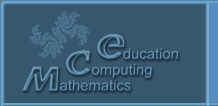Русский
 ! Вопросы и ответы

# Abstracts

## The associative distributive Algebras which are contravariat relatively the given quadratic isometry

Koganov A.V.

Scientific research institute of system analysis (FSE GSC NIISI RAN / FSE GSC SRISA RAS), Nakhimovsky st., 36, corp. 1, 117218, Moscow, Russia, koganow@niisi.msk.ru

1 pp. (accepted)

We solve the task of building the algebra with associative and distributive the addition and the product which is contravariant relative given quadratic form. Thous task arises at the quantum gravity problematic. We propose two algebras. 1. Bialgebra of matrix and vectors with scalar prodact. The medium is the all real matrix and vectors of given dimension. The operation are common summ and product of matrix, the summ of vectors, the act of the matrix on the vector and the special scalar product of two vectors wich gives the scalar matrix. 2) The algebra of matrix with the relation of metrix equivalence. The medium is the all real matrix. The operation are common summ and product of matrix. The addition binary relation of matrix is based on the special overdetermined system of equations. The relation selects the matrix pair which assumes the solution for that system. The teorem. The group of automorphism for tis algebras is the group of isometry for correspondly scalar product. The associativity and distributivity are inherited from the matrix algebra.

© 2004 Designed by Lyceum of Informational Technologies №1533